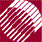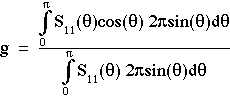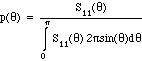ECE532 Biomedical Optics © 1998 Steven L. Jacques, Scott A. Prahl Oregon Graduate Institute

# Mie theory calculation of anisotropy (g)

Let's use the scattering pattern calculated previously for a 0.304-µm-dia. nonabsorbing polystyrene sphere in water irradiated by HeNe laser beam to calculate the anisotropy (g) of scattering.

The definition of anisotropy guides the calculation of g using the function S11(θ) which describes the scattered intensity for randomly polarized light:The denominator in the above equation assures proper normalization of p(θ):When numerically evaluating the above expression for g, a large number of angles need to be calculated, typically about 200 angles, in order to achieve a value of g with precision to at least 4 significant digits. For our example 0.304-µm sphere, the calculation of g based on S11(θ) yields g = 0.6608.

Note that the definition of g as cited in these notes assumes azimuthal symmetry, hence we have calculated the g for randomly polarized light. Due to the symmetry presented by a spherical particle, the g refers to scattering into all azimuthal angles regardless of the linear polarization of the incident light.